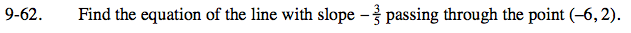### Home > CCA > Chapter 9 > Lesson 9.2.2 > Problem9-62

9-62.

Find the equation of the line with slope −passing through the point (−6, 2). Homework Help ✎Use the equation y = mx + b, where slope = m, to find the equation of the line. Solve for b.

$\textit{y}=-\frac{3}{5}\textit{x}-\frac{8}{5}$